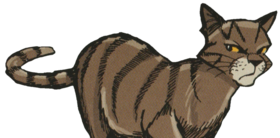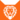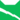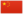—— 尘毛对黑莓星，关于成为长老，黑莓星的风暴》，第17页

Dustpelt《乌爪的旅程》狮族 (Q646)雷族 武士尘崽非官 Dustkit  小塵非官
 尘爪 Dustpaw  塵掌
 尘毛 Dustpelt  塵皮

Q718：雷族公猫，自《预言开始》系列登场的角色

## 细节

### 外貌

• 他是一只动作敏捷、:307身形沉重、:53琥珀色眼睛:58的暗棕色虎斑公猫，:猫物表有着泛灰的口鼻。:286

### 作者声明

• WarriorCats.com的家谱树中，他是绒毛和知更翅的儿子，樱桃爪、小板栗、霜毛、纹脸和乌爪的手足，长尾的异父手足。
• 基立证实他对火星冷嘲热讽是因为他喜欢沙风，但沙风后来爱上了火星。
• WarriorCats.com的文章〈脱颖而出〉中共选出了五组师徒，红尾和他排名第三。

### 勘误

• 他曾被错误地描述为淡棕色。:211
• 英国英文版的《风起云涌》中，他没有被列在猫物表上。:猫物表
• 他曾在成为武士后被错误地以学徒名称呼。:208
• 他的名字曾错误地拼写为“Dusltpelt”。:264

### 翻译

• 中文版的《天族与陌生者》附录的猫武士谱系图中，他以“尘掌”之名被列为武士。
• 正体中文版的《荒野手册》中，他曾不合惯例地被译为“尘毛”，[请求来源]详见惯例

## 语录

—— 尘爪对火心和灰条，《寒冰烈火》，第10页

—— 尘毛对虎掌，在红尾死亡的真相被揭发后，《疑云重重》，第276页

—— 狮焰对尘毛的印象，《战声渐近》，第230页

—— 冰云对尘毛，在香薇云死后，《鸽翅的沉默》，第1章

—— 尘毛对黑莓星，在他临死前，《黑莓星的风暴》，第288页

—— 黑莓星在尘毛死后想到，《黑莓星的风暴》，第288页

## 登场书目

• 呼唤野性 (Q57)[配角][首次登场🐱]
• 寒冰烈火 (Q59)[配角]
• 疑云重重 (Q60)[配角]
• 风起云涌 (Q65)[配角]
• 险路惊魂 (Q66)[配角]
• 力挽狂澜 (Q67)[配角]
• 午夜追踪 (Q139)[配角]
• 新月危机 (Q140)[配角]
• 重现家园 (Q141)[配角]
• 星光指路 (Q142)[配角]
• 黄昏战争 (Q143)[配角]
• 日落和平 (Q144)[配角]
• 预视力量 (Q152)[配角]
• 暗河汹涌 (Q153)[配角]
• 驱逐之战 (Q154)[配角]
• 天蚀遮月 (Q155)[配角]
• 暗夜长影 (Q156)[配角]
• 拂晓之光 (Q157)[配角]
• 第四学徒 (Q158)[配角]
• 战声渐近 (Q159)[配角]
• 暗夜密语 (Q160)[配角]
• 月光印记 (Q161)[配角]
• 武士归来 (Q162)[配角]
• 群星之战 (Q163)[配角]
• 学徒探索 (Q170)[对话提及]
• 火星的探索 (Q176)[配角]
• 蓝星的预言 (Q177)[配角]
• 黑莓星的风暴 (Q182)[配角][死亡时刻✝]
• 鸦羽的拷问 (Q186)[配角]
• 松鼠飞的希冀 (Q3561)[配角]
• 灰条的誓言 (Q4057)[对话提及]
• 冬青叶的故事 (Q187)[猫物闲角]
• 雾星的征兆 (Q188)[猫物闲角]
• 虎掌的愤怒 (Q190)[配角]
• 叶池的祈愿 (Q191)[对话提及]
• 鸽翅的沉默 (Q192)[配角]
• 乌爪的告别 (Q195)[对话提及]
• 红尾的恩债 (Q3565)[配角]
• 蛾翅的秘密 (Q3628)[猫物闲角]
• 黑脚的清算 (Q4336)[猫物闲角]
• 和平破碎 (Q213)[仅于回忆]
• 族群救星 (Q214)[配角]
• 河族阴云 (Q4311)[对话提及]
• 变革之风 (Q4411)[配角]
• 族群的秘密 (Q199)[追溯修订]
• 族群的猫 (Q200)[对话提及]
• 族群的守则 (Q201)[配角]
• 族群的战争 (Q202)[配角]
• 终极指南 (Q204)[对话提及]
• 族群决定 (Q4580)[配角]

## 参考文献

这篇文章基于CC BY-SA 3.0许可使用了猫武士维基（英语）Dustpelt一文中的部分内容。
0.0
0人评价